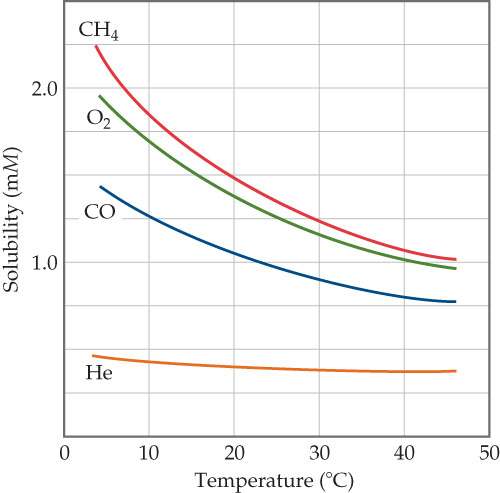# Problem: Solubilities of four gases in water as a function of temperature. The solubilities are in millimoles per liter of solution, for a constant total pressure of 1 atm in the gas phase.Where would you expect N2 to fit on this graph?

###### FREE Expert Solution

We have to determine where would the solubility of nitrogen gas (N2) lie on the given solubility-temperature curve.

The solubility of a gas in water is affected by various factors such as:

• Polarity of the gas
• Partial pressure of gas
89% (236 ratings)###### Problem DetailsSolubilities of four gases in water as a function of temperature. The solubilities are in millimoles per liter of solution, for a constant total pressure of 1 atm in the gas phase.

Where would you expect N2 to fit on this graph?